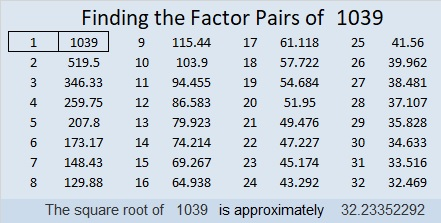# 1039 and Level 2

The eleven clues in this puzzle are enough to figure out where to place the factors and then complete the entire multiplication table. Try it. I know you can solve it!Print the puzzles or type the solution in this excel file: 10-factors-1035-1043

Now here’s a little about the number 1039:

• 1039 is a prime number.
• Prime factorization: 1039 is prime.
• The exponent of prime number 1039 is 1. Adding 1 to that exponent we get (1 + 1) = 2. Therefore 1039 has exactly 2 factors.
• Factors of 1039: 1, 1039
• Factor pairs: 1039 = 1 × 1039
• 1039 has no square factors that allow its square root to be simplified. √1039 ≈ 32.23352

How do we know that 1039 is a prime number? If 1039 were not a prime number, then it would be divisible by at least one prime number less than or equal to √1039 ≈ 32.2. Since 1039 cannot be divided evenly by 2, 3, 5, 7, 11, 13, 17, 19, 23, 29 or 31, we know that 1039 is a prime number.1039 is a palindrome when it is written in two different bases:
It’s 727 in BASE 12 because 7(144) + 2(12) + 7(1) = 1039, and
494 in BASE 15 because 4(225) + 9(15) + 4(1) = 1039

This site uses Akismet to reduce spam. Learn how your comment data is processed.